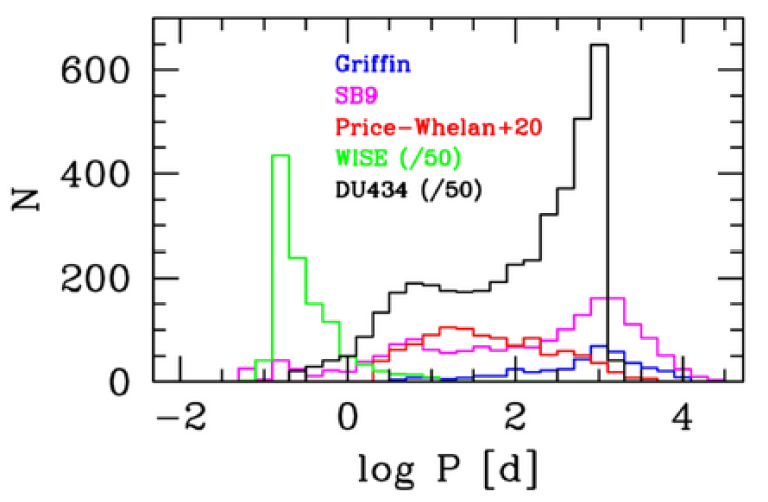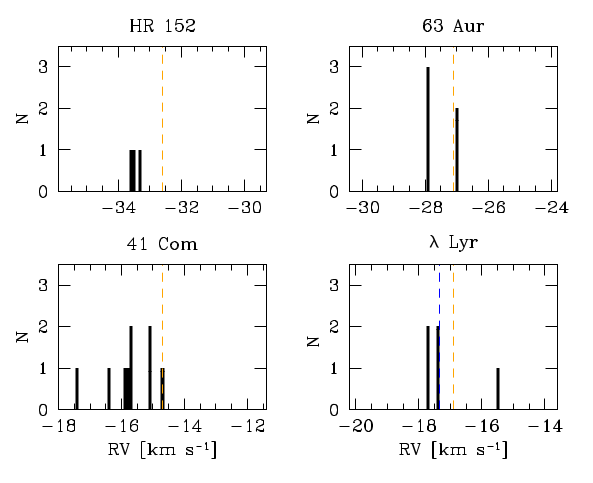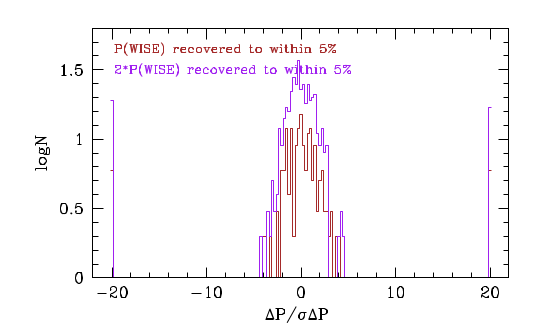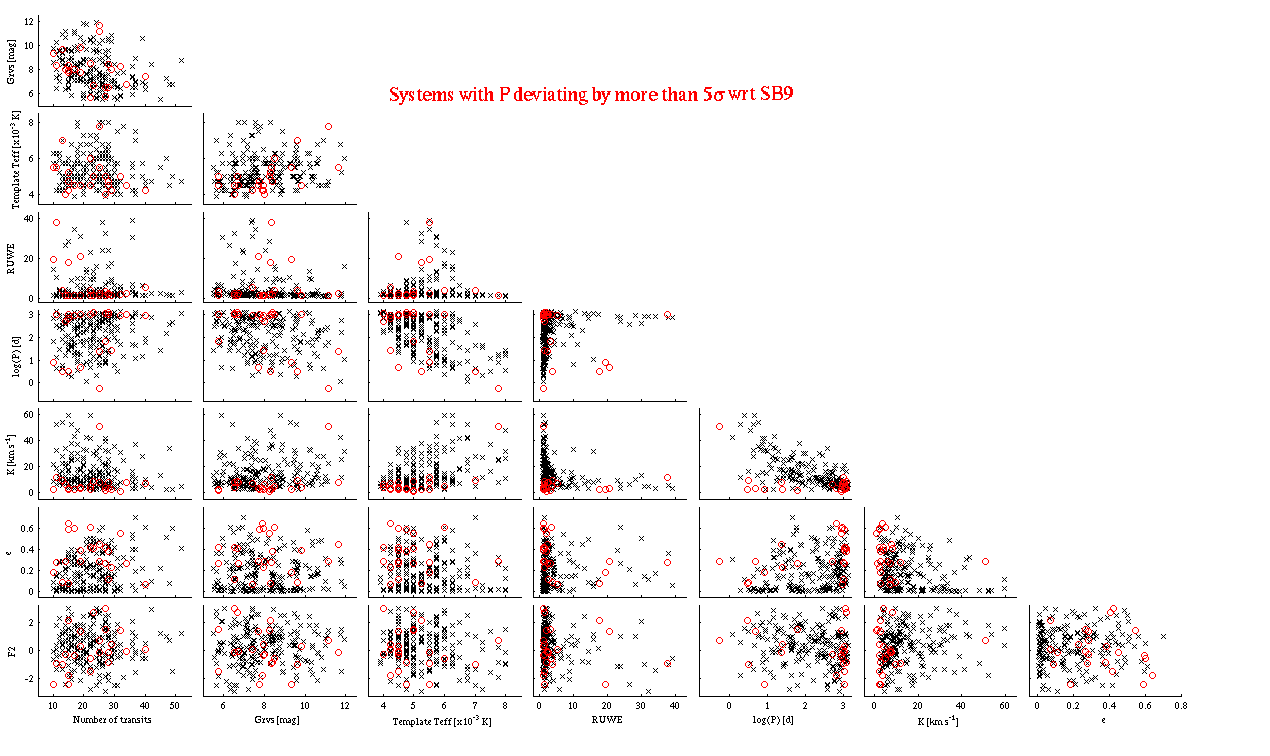# 7.4.6 Quality assessment and validation: validationFigure 7.30: Breakdown of the orbital periods for the Gaia DR3 SB (black), Griffin (blue), SB9 (magenta), APOGEE (red) and WISE (green) samples. The counts for Gaia DR3 SB and WISE have been divided by 50.

We present below a comparison between the Gaia DR3 orbital parameters and those from external catalogues (Griffin, SB9, APOGEE and WISE). The most distinctive feature distinguishing the various samples is the widely different range of orbital periods (Figure 7.30).

## Comparison against SB9 catalogue

A comparison was made between the Gaia DR3 orbital solutions based on the RVS spectroscopic processing data and those in a recent version (last updated 2021-03-02) of the SB9 catalogue (https://sb9.astro.ulb.ac.be/)

We first considered the orbits determined in a series of papers (not only in The Observatory) by Roger Griffin and collaborators. To avoid any ambiguities when cross-matching the SB9 and Gaia DR3 IDs, or a possible bias in centre-of-mass velocity, $\gamma$, visual/multiple systems were excluded. They were identified as having a component field in SB9 not empty or to have a SB9 ID entry in the 2020-06-23 version of the Multiple Star Catalog (MSC, Tokovinin 2018). This validation catalogue contains the orbits of 414 SB1’s and 101 SB2’s.

The breakdown of Gaia DR3 spectroscopic solution types is as follows for SB1:

• SB1: 111 systems

• SB1C: 0 system

• TrendSB1: 10 systems

• SB2: 2 systems

• SB2C: 0 system

• Without any Gaia DR3 deterministic solutions: 291 systems

The period of the 10 systems with a trend solution is much longer than the time span of the RVS observations, which is as expected.

The breakdown of Gaia DR3 spectroscopic solution types is as follows for SB2:

• SB2: 10 systems

• SB2C: 2 systems

• SB1: 7 systems

• SB1C: 0 system

• TrendSB1: 1 system

• Without any Gaia DR3 spectroscopic deterministic solutions: 81 systems

Figures 7.31, 7.32, 7.33 and 7.34 show the comparison between the Gaia DR3 and Griffin’s SB1 orbital parameters, as a function of various Gaia DR3 parameters. The difference is expressed as [value(Gaia)–value(Griffin)]/$\sigma$, where $\sigma$ is the square root of the sum in quadrature of both uncertainties. In the following, literature results without an uncertainty given are ignored. The deviations in $P$ expressed in $\sigma$ units are shown in Figure 7.35, as a function of the reference period. Systems for which the reference period, $P$, is difficult to find because of the limited length of the RVS observations (i.e. $\Delta T$ = time span observations $<$ $P$) are indicated with a different colour. Systems for which this period is not recovered to within 3$\sigma$ are also flagged.

Some deviating points can be noticed, especially when the period is not recovered. The incidence of outliers is quantified below. In some cases, the agreement is satisfactory in absolute terms and the deviation is only due to (very) small uncertainties. There is clear evidence for a systematic $\gamma$ offset, although the discrepancy only amounts to $\sim 1$ $\rm\,km\,s^{-1}$ on average. It might be due to the fact that the RV values adopted by Griffin for his four reference stars back in the late 60’s (Griffin 1969) appear to be slightly larger than those in the recent literature, including Soubiran et al. (2018b) for $\lambda$ Lyr (Figure 7.36).Figure 7.36: Comparison for the reference stars adopted by Griffin (1969) between his RVs and those in the more recent literature including Soubiran et al. (2018b). The literature data are shown as a histogram. The values of Griffin (1969) and Soubiran et al. (2018b) are shown as orange and blue dashed lines, respectively.

Table 7.2 summarises the recovery rate of the SB1 parameters to within 3$\sigma$ (only systems with uncertainties from Griffin are counted). Cases where the time span of the RVS observations is longer/shorter than the reference period are listed separately. As may be expected, the performance of the pipeline greatly improves when the observations cover more than one orbital cycle.

As a next step, the analysis above was repeated for the whole SB9. Although the sample is considerably larger, it is much less homogeneous and contains solutions of generally lower quality. The most recent orbit was selected when a given system had multiple entries in the catalogue. As described above, visual/multiple systems were also excluded. Six SB2 orbits were replaced by those determined by Lester (2020). This validation catalogue contains the orbits of 1750 SB1’s and 647 SB2’s.

The breakdown of Gaia DR3 spectroscopic solution types is as follows for SB1:

• SB1: 278 systems

• SB1C: 0 system

• TrendSB1: 47 systems

• SB2: 4 systems

• SB2C: 0 system

• Without any Gaia DR3 spectroscopic deterministic solutions: 1421 systems

All but one of the 47 systems with a trend solution have a very long period.

The breakdown of Gaia DR3 spectroscopic solution types is as follows for SB2:

• SB2: 91 systems

• SB2C: 14 systems

• SB1: 21 systems

• SB1C: 0 system

• TrendSB1: 2 systems

• Without any Gaia DR3 deterministic solutions: 519 systems

Figures 7.37, 7.38, 7.39 and 7.40 show the comparison between the orbital parameters. The deviations in $P$ expressed in $\sigma$ units are shown in Figure 7.41, as a function of the reference period.

The results are consistent with those obtained for Griffin’s sample. Some features are expected. For instance, the difficulty to find the correct period for (very) wide, low-amplitude systems. Once again, the large discrepancies may be misleading in some cases. For instance, some short-period systems are outliers in $P$ because the uncertainties are very small ($\sim$10${}^{-4}$ d). The same conclusion holds for SB2’s (Section 7.5.5). The slight systematic discrepancy and large dispersion for $\gamma$ is likely due to the fact that a significant fraction of these orbits are actually taken from Griffin (see discussion about offset above). The $\gamma$ deviations indeed show some evidence for a bimodal distribution (Figure 7.38).

Table 7.3 summarises the recovery rate of the SB1 parameters to within 3$\sigma$. Once again, not surprisingly, the incidence of outliers decreases when the time span of the observations exceeds one orbital cycle.

## Comparison against APOGEE

Orbital parameters for $\sim$20 000 SB1’s have been released as part of APO Galactic Evolution Experiment (APOGEE, http://www.sdss3.org/surveys/apogee.php) DR16 (Price-Whelan et al. 2020, hereafter PW20). The validation sample is their ”Gold Sample” that contains 1032 systems with high-quality, uni-modal posterior samplings.

The breakdown of Gaia DR3 spectroscopic solution types is as follows for SB1:

• SB1: 134 systems

• SB1C: 0 system

• TrendSB1: 5 systems

• SB2: 3 systems

• SB2C: 0 system

• Without any Gaia DR3 deterministic solutions: 890 systems

Four of the five systems with a trend solution have a period much longer than $\Delta T$.

Figures 7.42, 7.43, 7.44 and 7.45 show the comparison between the orbital parameters. The deviations in $P$ expressed in $\sigma$ units are shown in Figure 7.46, as a function of the reference period. For the period, two situations are encountered: either there is a reasonable correspondence or the values are widely different. It is cautioned that a significant number of systems in Figs. 7.45 and 7.46 are off scale because they belong to the second category.

Table 7.4 summarises the recovery rate of the SB1 parameters to within 3$\sigma$. The lower incidence of outliers when a full orbital cycle is not covered may be attributed to small number statistics.

## Comparison against WISE

A total of $\sim$56 000 short-period ($<$ 10 d) binary systems observed by the Wide-field Infrared Survey Explorer (WISE, https://www.nasa.gov/mission_pages/WISE/main/index.html) most of them being EBs, were discussed by Petrosky et al. (2021). A subset of 49 465 systems can be unambiguously associated with a Gaia DR3 source. This sample allows us to test the reliability of the orbital periods for close binaries, although other orbital parameters are not available.

We find a close correspondence in most cases with either the main WISE period or its second harmonic (Figure 7.47). As discussed by Petrosky et al. (2021), the orbital period they found is usually half the true value because of the nearly symmetrical nature of the WISE light curves. The WISE period is recovered to within 5% for 25.0% and 59.9% of the cases for the first and second harmonic, respectively. The overall recovery rate at this level is thus about 85%. It remains high for lower tolerances (e.g. $\sim$82% for 1%). The deviations lie within five times the mutual uncertainties (Figure 7.48).Figure 7.47: Ratio between spectroscopic Gaia DR3 and WISE periods. Gaia values that can be identified with the first and second WISE harmonics are indicated as vertical, dashed lines.Figure 7.48: Deviations in σ units between the Gaia DR3 and WISE periods when the latter (or its harmonic) are recovered to within 5%.

## SummaryFigure 7.49: Corner plot for the SB9 with the outliers in P highlighted in red.

As a preamble to summarising the main conclusions of our analysis, it is worth emphasising that some of the validation datasets discussed above are of limited usefulness in the sense that they do not offer stringent tests of the Gaia results. In particular, the APOGEE orbits (Price-Whelan et al. 2020) are based on fewer epochs (about 14 on average) than most NSS sources. As a consequence, their orbital solutions are not necessarily more robust and precise. It might be no coincidence that the highest recovery rates are found for the high-quality Griffin solutions (Table 7.2).

As discussed above, the success rate for the main orbital parameters ($P$, $K$, $e$ and $\gamma$) is usually well above 80% for the whole validation catalogues considered here (Griffin, SB9, APOGEE and WISE). The adopted criterion for an acceptable match is that the deviation lies within 3$\sigma$ of the quadratic sum of the random uncertainties. As expected, the performance increases for relatively short-period systems for which at least one orbital period is covered by the RVS observations (about 2.5 years for the RVS spectroscopic processing dataset considered here). In this case, the recovery rate commonly exceeds 90%. The WISE sample of close (eclipsing) binaries allows us to investigate the performance of our period search at high frequencies. The WISE photometric periods are recovered at a level of about 85%. The poor correspondence between the Gaia DR3 and Griffin/SB9 $\gamma$’s is ascribed to a slight zero-point offset at the $\sim 1$ $\rm\,km\,s^{-1}$ level. For the other parameters, no obvious systematic discrepancies are observed.

About 97% of the TrendSB1 solutions are associated with binaries with periods significantly longer than the observations. Conversely, although the proportion depends on the validation dataset, less than 10% of the SB1 solutions should not have received a solution of this type given the length of the observations.

Our ability to recover the orbital parameters quite significantly hinges on the retrieval of the true period. For instance, it is found that the recovery rate of the eccentricity typically increases by about 5% when the correct period is found. It is evident that other limitations, either related to the characteristics of the observations (e.g. number of RVS transits) or the very nature of the binary system (e.g. velocity semi-amplitude) also play a significant role in the quality of the solutions. Some clues about the sensitivity (or lack thereof) to the combination of some parameters can be gauged from plots such as Figure 7.49. However, these are complex, multi-dimensional dependencies that cannot be easily and reliably quantified given the quite limited number of cross matches with the validation catalogues (a few hundreds systems at most).